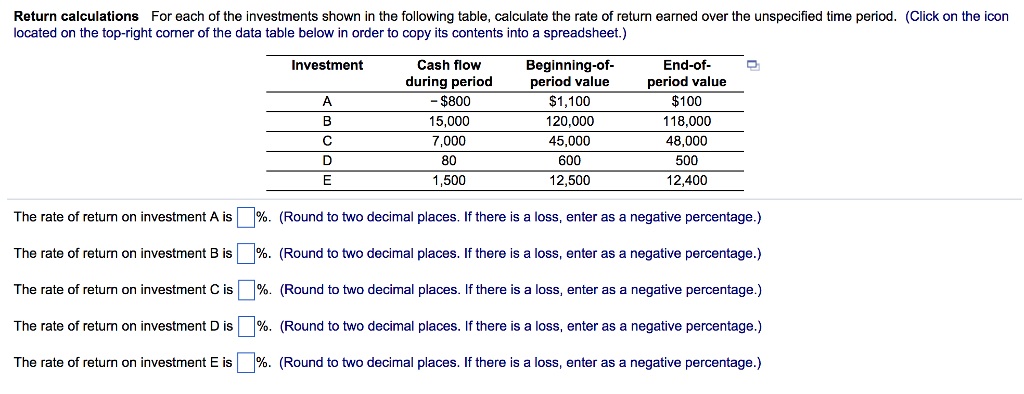### Create an Account

Home / Questions / Please answer all thank youFor each of the investments shown in the following For each of...

# Please answer all thank youFor each of the investments shown in the following For each of the investments shown in the following table calculate the rate of return earned over the unspecified time

Please answer all, thank you!For each of the investments shown in the following table, calculate the rate of return earned over the unspecified time period. (Click on the icon located on the top-right corner of the data table below in order to copy its contents into a spreadsheet.) The rate of return on investment A is %. (Round to two decimal places. If there is a loss, enter as a negative percentage.) The rate of return on investment B is %. (Round to two decimal places. If there is a loss, enter as a negative percentage.) The rate of return on investment C is %. (Round to two decimal places. If there is a loss, enter as a negative percentage.) The rate of return on investment D is %. (Round to two decimal places. If there is a loss, enter as a negative percentage.) The rate of return on investment E is %. (Round to two decimal places. If there is a loss, enter as a negative percentage.)

Apr 02 2020 View more View LessSubscribe To Get Solution